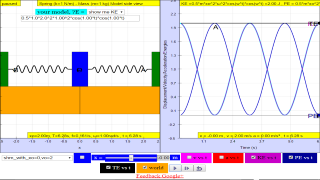SHM17

1.3 a)    Variation with time of energy in simple harmonic motion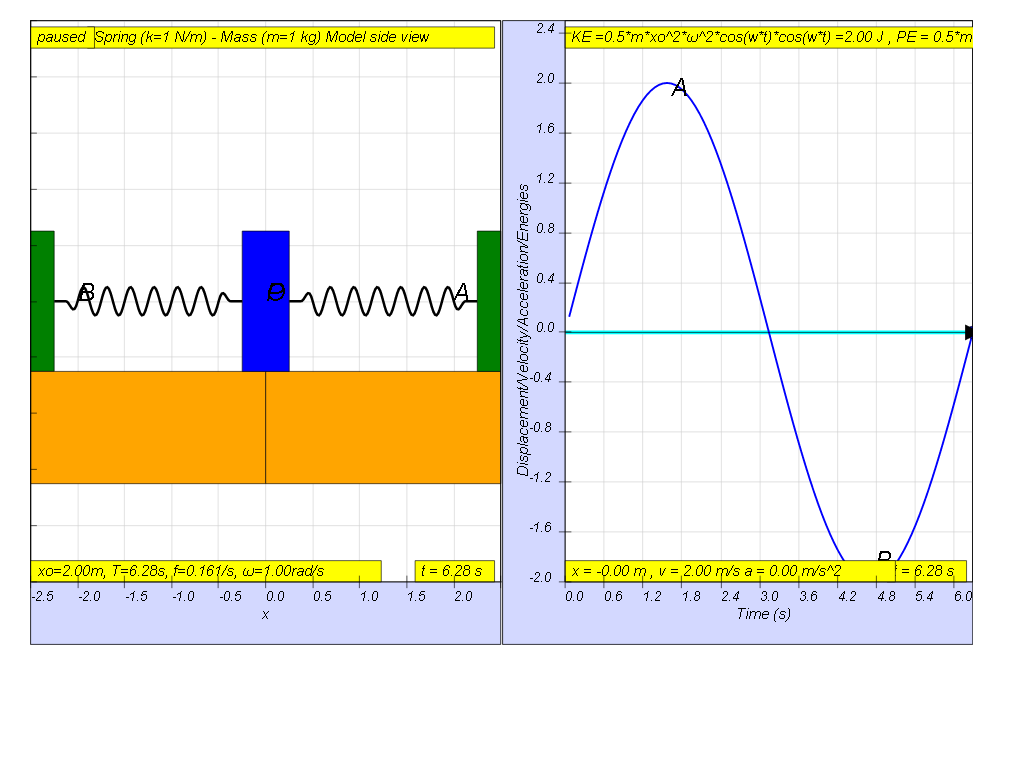If the variation with time of displacement is as shown, then the energies should be drawn as shown.

recalling Energy formula

KE = ½ m v2

PE =  ½ k x2

in terms of time t,

x = x0 sin(ωt)

differentiating with t gives

v = v0 cos (ωt)

therefore, KE = ½ m v2= ½ m (v0 cos (ωt))2= ½ m (x02ω2)cos (ωt))2

similarly

PE = ½ k x2= ½ (mω2 )(x0 sin (ωt))2= ½ m (x02ω2 )sin (ωt))2

therefore total energy is a constant value in the absence of energy loss due to drag (resistance)

TE = KE + PE = ½ m (x02ω2 )[cos2(ωt) + sin2(ωt))] = ½ m (x02ω2)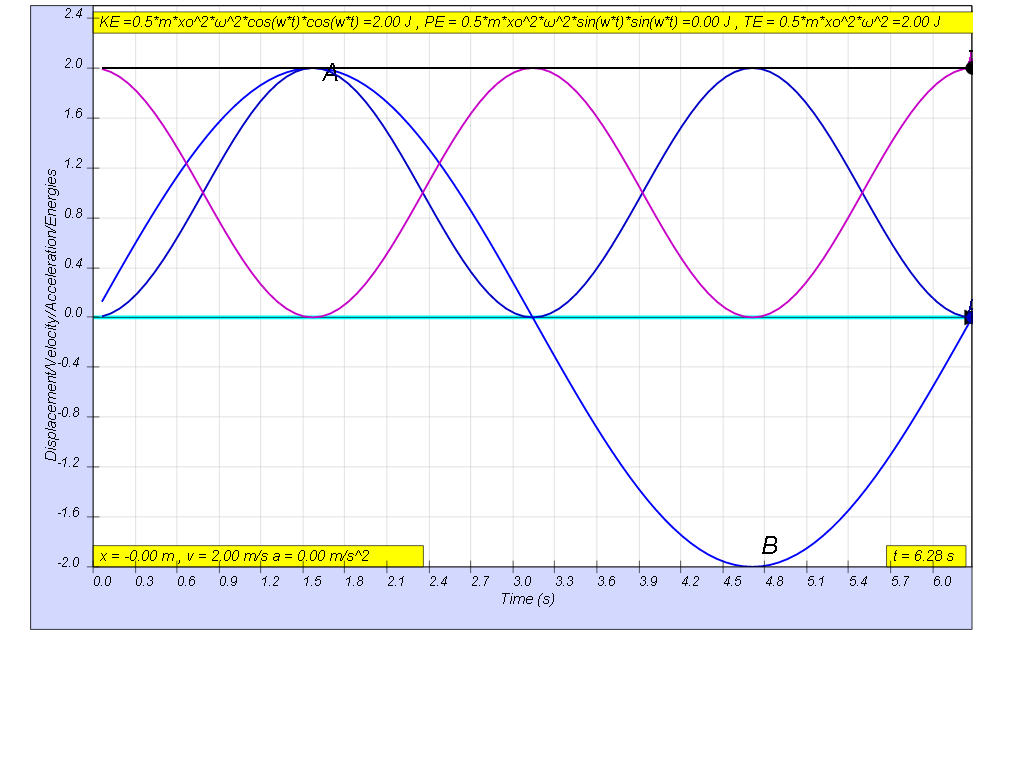this is how the x vs t looks together of the energy vs t graphs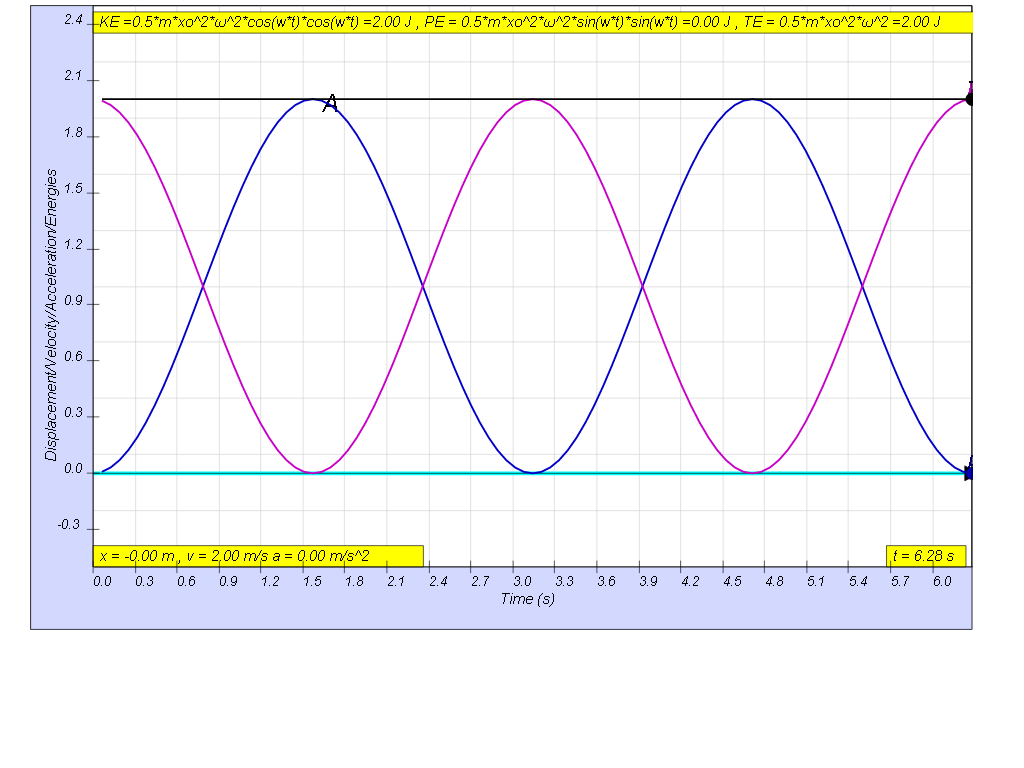1.3.1 Summary

the table shows some of the common values
 general energy formula SHM energy formula when t = 0 when t = T/4 when t = T/2 when t = 3T/4 when t = T KE = ½ m v2 ½ m (x02ω2)cos (ωt))2 ½ m (x02ω2) 0 ½ m (x02ω2) 0 ½ m (x02ω2) PE =  ½ k x2 ½ m (x02ω2)cos (ωt))2 0 ½ m (x02ω2) 0 ½ m (x02ω2) 0 TE = KE + PE TE = ½ m (x02ω2) ½ m (x02ω2) ½ m (x02ω2) ½ m (x02ω2) ½ m (x02ω2) ½ m (x02ω2)

Translations

Code Language Translator RunSoftware Requirements

SoftwareRequirements

 Android iOS Windows MacOS with best with Chrome Chrome Chrome Chrome support full-screen? Yes. Chrome/Opera No. Firefox/ Samsung Internet Not yet Yes Yes cannot work on some mobile browser that don't understand JavaScript such as..... cannot work on Internet Explorer 9 and below

CreditsThis email address is being protected from spambots. You need JavaScript enabled to view it.

end faq

http://iwant2study.org/lookangejss/02_newtonianmechanics_8oscillations/ejss_model_SHM17/SHM17_Simulation.xhtml

Apps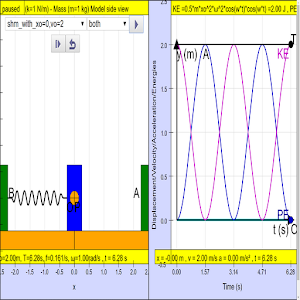https://play.google.com/store/apps/details?id=com.ionicframework.shm17app307408&hl=en

Other resources

https://ggbm.at/pY4Hvugh

end faqRating 5.00 (3 Votes)